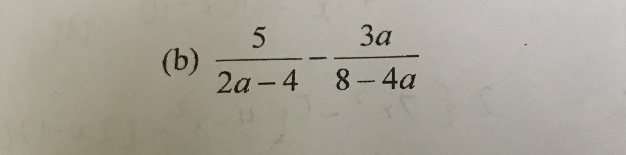# QuestionTia!

This is a Fractional algebraic expression.

5 / 2a – 4    –   3a / 8 – 4a

= 5 / 2(a – 2)    –  3a / 4(2 – a)      <——- Cousin  (a – 2 ) and (2 – a)

= 5/ 2(a – 2)   +   3a / 4(a – 2)      <——- change to a – 2

= 10 / 4(a – 2) +   3a / 4(a – 2)

= 10 + 3a   /   4(a – 2)

0 Replies 1 Like ✔Accepted Answer